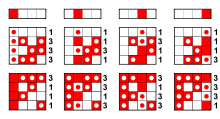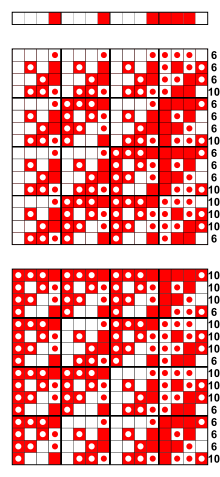# Bent function

﻿
Bent functionThe 2-ary bent functions with Hamming weight 1

Their nonlinearity is$2^{2-1} - 2^{\frac{2}{2}-1} = 2-1 = 1$The nonlinearity of the bent function$x_1 x_2~+~x_3 x_4$ is$2^{4-1} - 2^{\frac{4}{2}-1} = 8-2 = 6$$~+~$ stands for the exclusive or
(compare algebraic normal form)

In the mathematical field of combinatorics, a bent function is a special type of Boolean function. Defined and named in the 1960s by Oscar Rothaus in research not published until 1976, bent functions are so called because they are as different as possible from all linear and affine functions. They have been extensively studied for their applications in cryptography, but have also been applied to spread spectrum, coding theory, and combinatorial design. The definition can be extended in several ways, leading to different classes of generalized bent functions that share many of the useful properties of the original.

## Walsh transform

Bent functions are defined in terms of the Walsh transform. The Walsh transform of a Boolean function ƒ: Zn
2
Z2
is the function$\hat{f}:\Z_2^n \to \Z$ given by$\hat{f}(a) = \sum_{\scriptstyle{x \in \Z_2^n}} (-1)^{f(x) + a \cdot x}$

where a · x = a1x1 + a2x2 + … + anxn (mod 2) is the dot product in Zn
2
. Alternatively, let S0(a) = { xZn
2
: ƒ(x) = a · x }
and S1(a) = { xZn
2
: ƒ(x) ≠ a · x }
. Then |S0(a)| + |S1(a)| = 2n and hence$\hat{f}(a) = |S_0(a)| - |S_1(a)| = 2 |S_0(a)| - 2^n.$

For any Boolean function ƒ and aZn
2
the transform lies in the range$-2^n \leq \hat{f}(a) \leq 2^n.$

Moreover, the linear function ƒ0(x) = a · x and the affine function ƒ1(x) = a · x + 1 correspond to the two extreme cases, since$\hat{f}_0(a) = 2^n,~ \hat{f}_1(a) = -2^n.$

Thus, for each aZn
2
the value of$\hat{f}(a)$ characterizes where the function ƒ(x) lies in the range from ƒ0(x) to ƒ1(x). Bent functions are in a sense equidistant from all of these, so they are equally hard to approximate with any affine function.

## Definition and properties

Rothaus defined a bent function as a Boolean function ƒ: Zn
2
Z2
whose Walsh transform has constant absolute value.

The simplest examples of bent functions, written in algebraic normal form, are F(x1,x2) = x1x2 and G(x1,x2,x3,x4) = x1x2 + x3x4. This pattern continues: x1x2 + x3x4 + ... + xn − 1xn is a bent function Zn
2
Z2
for every even n, but there is a wide variety of different types of bent functions as n increases. The sequence of values (−1)ƒ(x), with xZn
2
taken in lexicographical order, is called a bent sequence; bent functions and bent sequences have equivalent properties. In this ±1 form, the Walsh transform is easily computed as$\hat{f}(a) = W(2^n) (-1)^{f(a)},$

where W(2n) is the natural-ordered Walsh matrix and the sequence is treated as a column vector.

Rothaus proved that bent functions exist only for even n, and that for a bent function ƒ,$|\hat{f}(a)|=2^{n/2}$ for all aZn
2
. In fact,$\hat{f}(a)=2^{n/2}(-1)^{g(a)}$, where g is also bent. In this case,$\hat{g}(a)=2^{n/2}(-1)^{f(a)}$, so ƒ and g are considered dual functions.

Every bent function has a Hamming weight (number of times it takes the value 1) of 2n − 1 ± 2n/2 − 1, and in fact agrees with any affine function at one of those two numbers of points. So the nonlinearity of ƒ (minimum number of times it equals any affine function) is 2n − 1 − 2n/2 − 1, the maximum possible. Conversely, any Boolean function with nonlinearity 2n − 1 − 2n/2 − 1 is bent. The degree of ƒ in algebraic normal form (called the nonlinear order of ƒ) is at most n/2 (for n > 2).

Although bent functions are vanishingly rare among Boolean functions of many variables, they come in many different kinds. There has been detailed research into special classes of bent functions, such as the homogeneous ones or those arising from a monomial over a finite field, but so far the bent functions have defied all attempts at a complete enumeration or classification.

## Applications

As early as 1982 it was discovered that maximum length sequences based on bent functions have cross-correlation and autocorrelation properties rivalling those of the Gold codes and Kasami codes for use in CDMA. These sequences have several applications in spread spectrum techniques.

The properties of bent functions are naturally of interest in modern digital cryptography, which seeks to obscure relationships between input and output. By 1988 Forré recognized that the Walsh transform of a function can be used to show that it satisfies the Strict Avalanche Criterion (SAC) and higher-order generalizations, and recommended this tool to select candidates for good S-boxes achieving near-perfect diffusion. Indeed, the functions satisfying the SAC to the highest possible order are always bent. Furthermore, the bent functions are as far as possible from having what are called linear structures, nonzero vectors a such that ƒ(x+a) + ƒ(x) is a constant. In the language of differential cryptanalysis (introduced after this property was discovered) the derivative of a bent function ƒ at every nonzero point a (that is, ƒa(x) = ƒ(x+a) + ƒ(x)) is a balanced Boolean function, taking on each value exactly half of the time. This property is called perfect nonlinearity.

Given such good diffusion properties, apparently perfect resistance to differential cryptanalysis, and resistance by definition to linear cryptanalysis, bent functions might at first seem the ideal choice for secure cryptographic functions such as S-boxes. Their fatal flaw is that they fail to be balanced. In particular, an invertible S-box cannot be constructed directly from bent functions, and a stream cipher using a bent combining function is vulnerable to a correlation attack. Instead, one might start with a bent function and randomly complement appropriate values until the result is balanced. The modified function still has high nonlinearity, and as such functions are very rare the process should be much faster than a brute-force search. But functions produced in this way may lose other desirable properties, even failing to satisfy the SAC—so careful testing is necessary. A number of cryptographers have worked on techniques for generating balanced functions that preserve as many of the good cryptographic qualities of bent functions as possible.

Some of this theoretical research has been incorporated into real cryptographic algorithms. The CAST design procedure, used by Carlisle Adams and Stafford Tavares to construct the S-boxes for the block ciphers CAST-128 and CAST-256, makes use of bent functions. The cryptographic hash function HAVAL uses Boolean functions built from representatives of all four of the equivalence classes of bent functions on six variables. The stream cipher Grain uses an NLFSR whose nonlinear feedback polynomial is, by design, the sum of a bent function and a linear function.

## Generalizations

The most common class of generalized bent functions is the mod m type,$f:\mathbb{Z}_m^n \to \mathbb{Z}_m$ such that$\hat{f}(a) = \sum_{x \in \mathbb{Z}_m^n} e^{\frac{2 \pi i}{m} (f(x) - a \cdot x)}$

has constant absolute value mn/2. Perfect nonlinear functions$f:\mathbb{Z}_m^n \to \mathbb{Z}_m$, those such that for all nonzero a, ƒ(x+a) − ƒ(a) takes on each value mn − 1 times, are generalized bent. If m is prime, the converse is true. In most cases only prime m are considered. For odd prime m, there are generalized bent functions for every positive n, even and odd. They have many of the same good cryptographic properties as the binary bent functions.

Semi-bent functions are an odd-order counterpart to bent functions. A semi-bent function is$f:\mathbb{Z}_m^n \to \mathbb{Z}_m$ with n odd, such that$|\hat{f}|$ takes only the values 0 and m(n+1)/2. They also have good cryptographic characteristics, and some of them are balanced, taking on all possible values equally often.

The partially bent functions form a large class defined by a condition on the Walsh transform and autocorrelation functions. All affine and bent functions are partially bent. This is in turn a proper subclass of the plateaued functions.

The idea behind the hyper-bent functions is to maximize the minimum distance to all Boolean functions coming from bijective monomials on the finite field GF(2n), not just the affine functions. For these functions this distance is constant, which may make them resistant to an interpolation attack.

Other related names have been given to cryptographically important classes of functions Zn
2
Zn
2
, such as almost bent functions and crooked functions. While not Boolean functions themselves, these are closely related to the bent functions and have good nonlinearity properties.

Wikimedia Foundation. 2010.

### Look at other dictionaries:

• bent crumpled dented — damaged damaged (d[a^]m [asl]jd), adj. 1. changed so as to reduce value, function, or other desirable trait; usually not used of persons. Opposite of {undamaged}. [Narrower terms: {battered, beat up, beaten up, bedraggled, broken down,… …   The Collaborative International Dictionary of English

• Ellis Bent — (1783 – 10 November 1815) was the deputy judge advocate between 1810 and 1815 of the Australian colony of New South Wales, which was eventually to become an Australian state. The deputy judge advocate was the senior legal officer of the colony… …   Wikipedia

• hash function — maišos funkcija statusas T sritis informatika apibrėžtis Funkcija, iš duomenų ↑įrašo arba jo ↑rakto (1), apskaičiuojanti ↑maišos reikšmę. Funkcijos algoritmas priklauso nuo to, kam bus naudojama jos reikšmė. Pavyzdžiui, jeigu projektuojama įrašų… …   Enciklopedinis kompiuterijos žodynas

• CAST-128 — The following article is about the block cipher. For the axion observatory in Switzerland, see CERN Axion Solar Telescope. Infobox block cipher name = CAST 128 caption = Three rounds of the CAST 128 block cipher designers = Carlisle Adams and… …   Wikipedia

• Jennifer Seberry — Jennifer Roma Seberry is a cryptographer, mathematician, and computer scientist, currently a professor at the University of Wollongong, Australia. She was formerly thehead of the Department of Computer Science and director of the Centre for… …   Wikipedia

• dance — dancingly, adv. /dans, dahns/, v., danced, dancing, n. v.i. 1. to move one s feet or body, or both, rhythmically in a pattern of steps, esp. to the accompaniment of music. 2. to leap, skip, etc., as from excitement or emotion; move nimbly or… …   Universalium

• hand tool — any tool or implement designed for manual operation. * * * Introduction  any of the implements used by craftsmen in manual operations, such as chopping, chiseling, sawing, filing, or forging. Complementary tools, often needed as auxiliaries to… …   Universalium

• epistemology — epistemological /i pis teuh meuh loj i keuhl/, adj. epistemologically, adv. epistemologist, n. /i pis teuh mol euh jee/, n. a branch of philosophy that investigates the origin, nature, methods, and limits of human knowledge. [1855 60; < Gk… …   Universalium

• international relations — a branch of political science dealing with the relations between nations. [1970 75] * * * Study of the relations of states with each other and with international organizations and certain subnational entities (e.g., bureaucracies and political… …   Universalium

• LSm — s.The Sm proteins were first discovered as antigens in a patient with a form of systemic lupus erythematosus (SLE), a debilitating autoimmune disease. They were named Sm proteins in honor of this patient, Stephanie Smith. Other proteins with very …   Wikipedia